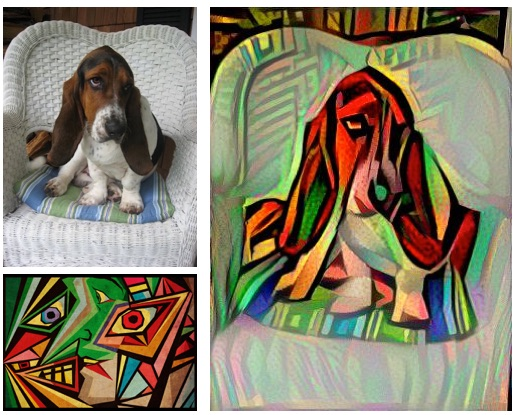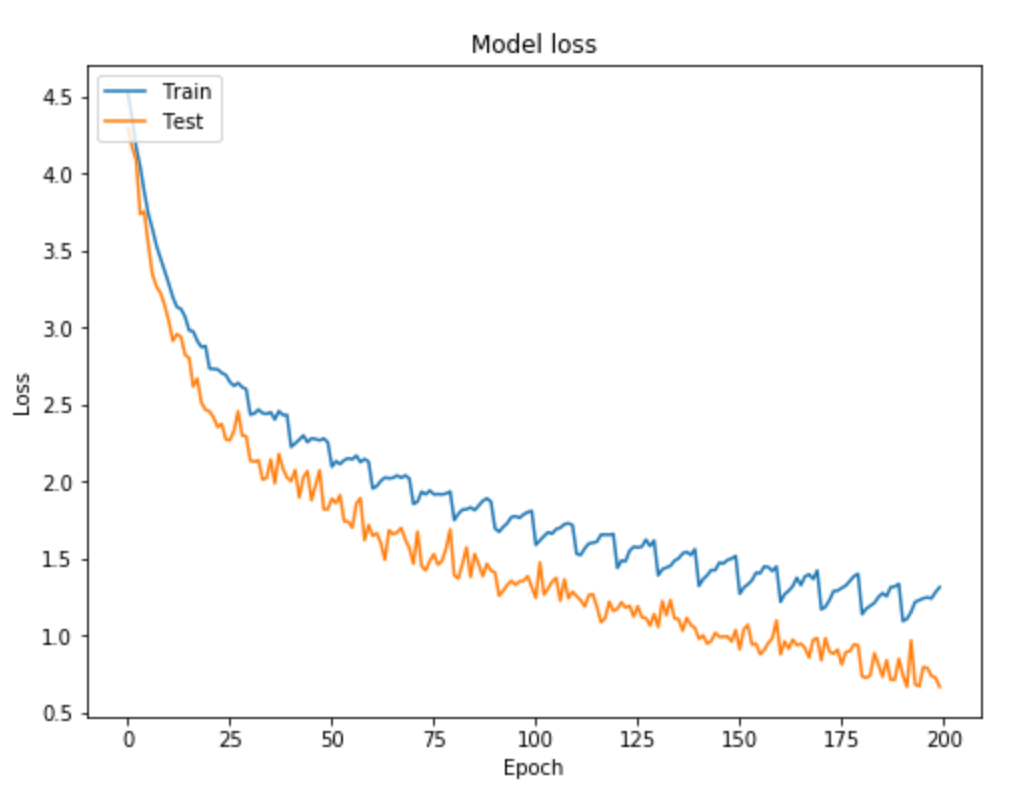﻿ Neural Style Transfer

## Neural Style Transfer.#### What is Neural Style Transfer?

Neural Style Transfer is a method of combining two images together. One as a base, and one as the style. An example would be taking a picture of your dog and a picture of the famous paints "Starry Night", and combining them to make it look as if your dog was painted by Van Gogh in the style of Starry Night.

#### Convolutional Neural Networks

Well, first off, CNN's are different from a regular neural network as they don't sequentially go through data (pixels in this case) like most neural networks do. Instead, they look at multiple pixels at once, almost like sliding a filter over the image.

The layers of a CNN are made of mostly convolutional layers (hence the name) which help create "features", and each layer is progressively more complex. Here is a visualization:

The filter here is the red numbers we are multiplying the yellow numbers against. Basically, we look at one part of the image, apply the filter, and sum the result to get a convolved feature. If we think of the image being grayscale, each of the original numbers is how bright that pixel is.#### Pooling Layers

Pooling is much simpler and also similar to convolution. Instead of detecting features, pooling is meant to lighten the load on the network by reducing its spatial size. Basically, it reduces the size of the inputs of the convolutional layers, with generally two methods:

Max Pooling
Max pooling is the first type, wherein you take the biggest number in each cell of the image.

Average Pooling
The second type of pooling, wherein you average all the numbers in each cell of the image.

#### Activation Layers

Activation layers are usually key for a neural network. It allows us to make the final prediction.

However, here we won't be making a prediction, and we will only be using convolutional (and pooling) layers. What we will do is create a loss function, which will be what blends the image and will also allow us to control how it is blended.#### Loss Functions

The loss function will be essentially how far off the neural network is from the original image, and this loss function will actually be made of two different loss functions that make up a "total" loss function. We want to minimize the loss of the function, basically meaning we want it to be close to the original image.

The two loss functions I mentioned before are for the two images we are going to input, the content image, and the style image, which we want to apply to the content image. Then we can combine those with some weights (which we can change to tweak how much style/content comes out), and then each other, to get our loss function.

#### Optimizers

I did kind of lie before however because I said that WE tweak the weights for the loss function. The computer will actually do the tweaking for us, with an optimizer. This optimizer will essentially try it's best to lower the total loss function that was explained earlier, and after it does that, we are done!

Of course, we don't actually feed an image into the neural network, we simply turn that image into data in an array, and then feed that into the neural network. Afterward, we have to turn the data the network spits out into our new styled image!## contact me.

Feel Free to Contact